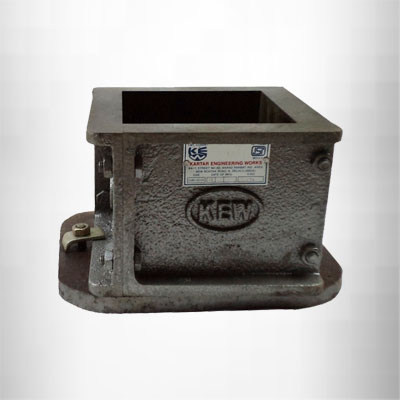## Mould

Mould for 5 cm cube with base plate, made of Cast Iron, as per IS : 4031 (Part-I), IS :10080-1982

1. Cast Iron Mould for 7.06 cm cube, with loose base plate with "ISI" Mark, as per IS : 4031, IS : 10080-1982.
2. Cast Iron Mould for 10 cm cube, Cast Iron with base plate, with "ISI" Mark, IS : 516, IS : 10086 - 1982.
3. Cast Iron Mould for 15 cm cubes, with loose base plates. IS : 516, IS : 10086 - 1982, with "ISI" Mark.
4. Cast Iron Mould for 20 cm cubes, as per IS : 516, IS : 10086 - 1982
5. Cast Iron Mould for 30 cm cubes, as per IS : 516, IS : 10086 - 1982

Category:

Mould for 5 cm cube with base plate, made of Cast Iron, as per IS : 4031 (Part-I), IS :10080-1982

1. Cast Iron Mould for 7.06 cm cube, with loose base plate with “ISI” Mark, as per IS : 4031, IS : 10080-1982.
2. Cast Iron Mould for 10 cm cube, Cast Iron with base plate, with “ISI” Mark, IS : 516, IS : 10086 – 1982.
3. Cast Iron Mould for 15 cm cubes, with loose base plates. IS : 516, IS : 10086 – 1982, with “ISI” Mark.
4. Cast Iron Mould for 20 cm cubes, as per IS : 516, IS : 10086 – 1982
5. Cast Iron Mould for 30 cm cubes, as per IS : 516, IS : 10086 – 1982